# Circle - simple

The circumference of a circle is 198 mm. How long in mm is its diameter?

D =  63.03 mm

### Step-by-step explanation: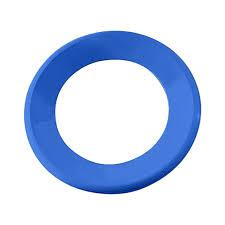Did you find an error or inaccuracy? Feel free to write us. Thank you!#### You need to know the following knowledge to solve this word math problem:

We encourage you to watch this tutorial video on this math problem:

## Related math problems and questions:

• ShapePlane shape has a maximum area 677 mm2. Calculate its perimeter if perimeter is the smallest possible.Calculate the radius of the circle whose length is 107 cm larger than its diameter
• Circle from string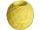Martin has a long 628 mm string. He makes a circle from it. Calculate the radius of the circle.
• CircleHow big is an area of a circle if its circumference is 51.2 cm?
• The parallelogram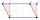The parallelogram has one side 2 dm long, which is one-sixth of its circumference. How many cm does the other side of the parallelogram measure?
• Simple equation 2Find X in this simple equation: X/9 = 96/108
• Isosceles triangleThe circumference of the isosceles triangle is 32.5 dm. Base length is 153 cm. How long is the leg of this triangle?
• V-belt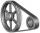Calculate the length of the belt on pulleys with diameters of 105 mm and 393 mm at shaft distance 697 mm.
• Engine pulley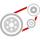The engine has a 1460 rev / min (RPM). Disc diameter is 350 mm. What will be the disc peripheral speed in RPM? Pulleys on the engine has diameter 80mm, on a disc has diameter 160mm.
• Four circles1) Calculate the circle radius if its area is 400 cm square 2) Calculate the radius of the circle whose circumference is 400 cm. 3) Calculate circle circumference if its area is 400 cm square 4) Calculate the circle's area if perimeter 400 cm.
• Running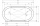The length of the inner edge of the running oval is 400 m. The straight sections measure 90 m. Calculate the dimensions of the oval - the rectangle where this oval can fit.
• The coilHow many ropes (the diameter 8 mm) fit on the coil (threads are wrapped close together) The coil has dimension: the inner diameter 400mm, the outside diameter 800mm and the length of the coil is 470mm
• Second sideCalculate the length of the other side of the rectangle if its circumference is 60 cm and one side is 10 cm long.
• 6-gon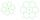Perimeter of regular hexagon is 113. Calculate its circumradius (radius of circumscribed circle).
• Circle simpleCircumference of a circle is 6.28. What is the area of the circle?
• Intersections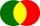How many intersections have circles with radius 16 mm and 15 mm, if the distance of their centers is 16 mm.
• QuotientFind quotient before the bracket - the largest divisor 51 a + 34 b + 68 121y-99z-33